#### You may also like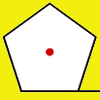### How Far Does it Move?

Experiment with the interactivity of "rolling" regular polygons, and explore how the different positions of the red dot affects the distance it travels at each stage.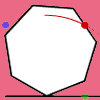### Up and Across

Experiment with the interactivity of "rolling" regular polygons, and explore how the different positions of the dot affects its vertical and horizontal movement at each stage.Logo helps us to understand gradients of lines and why Muggles Magic is not magic but mathematics. See the problem Muggles magic.

# Lying and Cheating

##### Age 11 to 14Challenge Level

Follow the instructions and you can take a rectangle, cut it into 4 pieces, discard two small triangles, put together the remaining two pieces and end up with a rectangle the same size. Try it! Clearly there is something wrong here but can you explain what it is?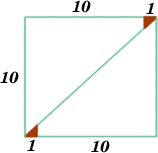-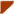-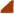=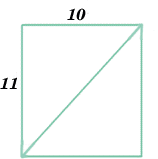Take some squared paper and cut out a rectangle 10 units by 11 units. As shown in the diagram, cut along the diagonal and cut off the two red triangles, each of which has a side of length one unit. Put the two red triangles to one side.

Now slide the right hand piece down (moving one edge that had been the diagonal along the other) until you make another rectangle. The top edge of the new rectangle is 10 units long but the left hand edge becomes 11 units. Thus the new rectangle is the same as the one you started with. (11 units by 10 units).